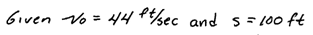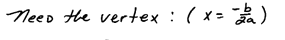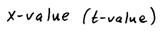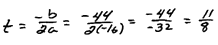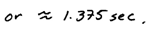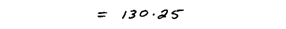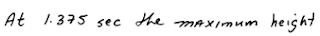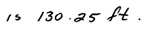## Pages

### Graphing Parabolas

At this point in our study we should be able to find x- and y-intercepts and solve any quadratic equation. Now we will learn an easy method used to graph them.

The graph of a quadratic equation is called a parabola.One of our basic functionscan be graphed by plotting points. We do this by choosing about five x-values and finding their corresponding y-values.

Graph:The more points we plot the easier it is to see that the graph is u-shaped. The vertex, in this case, is the point where the graph changes from decreasing to increasing, or the point with the smallest y-value. Here the vertex is (0, 0) which is also the x- and y-intercept. The line x = 0, the y-axis, is the line of symmetry. This is the line where we could fold our paper to see that the two sides of the graph coincide.
Given the graph find the x- and y-intercepts, vertex, a 5th point on the graph and the line of symmetry.Line of symmetry: x = 1
x-intercepts: (-2,0) and (4,0)
y-intercept: (0, -8)
Vertex: (1, -9)
5th point: (2, -8)

Recall that two points determine a line - this is not the case for parabolas. Parabolas require a minimum of 3 points but we usually want to find at least five points to make a nice graph. Find the vertex, x- and y- intercepts as well as the line of symmetry.Graph:Step 1: Find the y-intercept, (0, c).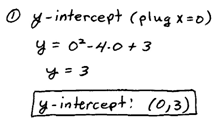Step 2: Find the x-intercepts by setting y = 0 and solving for x.Step 3: Find the vertex. You can find the x-value of the vertex using the vertex x = -b/(2a).Step 4: Graph the points and identify the axis of symmetry.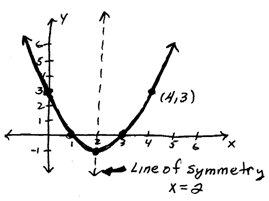The domain and range of the above function can be determined by the graph. In the previous problem the domain consists of all real numbers and the range consists of all real numbers greater than or equal to −1. Also, it is useful to note that we have a minimum y-value of −1, this will be an important fact when working the word problems.

Tip: The axis of symmetry of any quadratic function will be the vertical line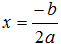When trying to find x-intercepts where the resulting quadratic equation does not factor, simply use the quadratic formula to solve it.

Graph: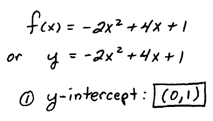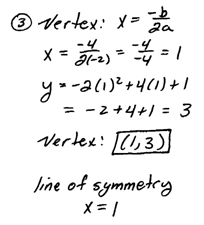This parabola looks a bit different, notice that it opens down and also notice that the previous parabola opened up. There is an easy test to know which way it opens even before we begin.Therefore, when asked to graph a parabola, you can get two important pieces of information without doing any work. By inspection you can tell if it opens up or down and you can identify y-intercept.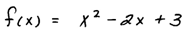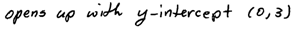Graph and label all important points: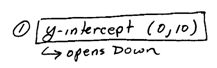Graph and label all important points: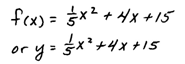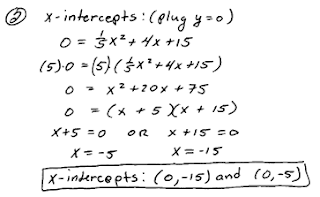The domain of the previous problem is all real numbers and the range consists of all real numbers greater than or equal to −5. Also, make a note that the minimum y-value is −5. It turns out that not all parabolas have two x-intercepts as we would expect. Sometimes they have only one x-intercept and sometimes none.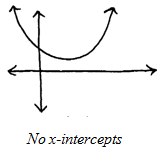Please keep in mind that all quadratic functions have a vertex and a y-intercept. In addition, we will still be able to find another point using symmetry. So in some cases it is acceptable to plot and label only three points.

Graph and label all important points: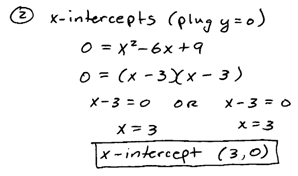Graph and label all important points: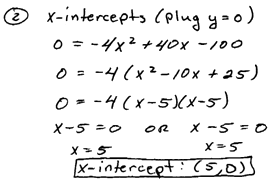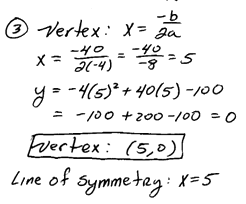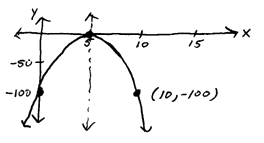Projectile Problem: An object is thrown from a 100 foot building at an initial speed of 44 feet per second. How long will it take to reach the maximum height? What is the maximum height?